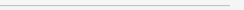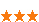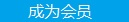您可以捐助，支持我们的公益事业。 1元 10元 50元 认证码：必填求知 文章 文库 Lib 视频 iProcess 课程 认证 咨询 工具 讲座 Modeler Code要资料订阅捐助
java中的基本算法319 次浏览     评价： 好 中 差
2019-5-15

 编辑推荐: 本文来自于cnblogs，本文主要介绍了java中的链表、二叉树以及冒泡排序、快速排序等几种排序方法，希望对大家能有所帮助。

1.链表

 public class Node{ private Object data; private Node next; public Node(Object data){ this.data = data; } //省略set、get方法 }

2.二叉树

 public class BinTree { private BinTree lChild;//左结点 private BinTree rChild;//右结点 private Object data; //数据域 public BinTree getlChild() { return lChild; } public void setlChild(BinTree lChild) { this.lChild = lChild; } public BinTree getrChild() { return rChild; } public void setrChild(BinTree rChild) { this.rChild = rChild; } public Object getData() { return data; } public void setData(Object data) { this.data = data; } }

3.排序

（1）冒泡排序

 public static void bubbleSort(int []arr) { for(int i =0;iarr[j+1]) { //把大的数放在后面 int temp = arr[j]; arr[j]=arr[j+1]; arr[j+1]=temp; } } } }

（2）快速排序

 public static void quickSort(int[] numbers,int low,int high){ if(low < high) { 　　int middle = getMiddle(numbers,low,high); //将numbers数组进行一分为二 　　quickSort(numbers, low, middle-1); //对低字段表进行递归排序 　　quickSort(numbers, middle+1, high); //对高字段表进行递归排序 } }

（3）选择排序

 public static void selectSort(int[]a){ int minIndex=0; int temp=0; for(int i=0;i

（4）插入排序

 private static int[] insertSort(int[]arr){ for(int i=1;i0;j--){ if(arr[j]

（5）希尔排序

 public static void main(String [] args) { int[]a={49,38,65,97,76,13,27,49,78,34,12,64,1}; //希尔排序 int d=a.length; while(true){ d=d/2; for(int x=0;x=0&&a[j]>temp;j=j-d){ a[j+d]=a[j]; } a[j+d]=temp; } } if(d==10){ break; } } }

（6）归并排序

 public static int[] sort(int[] nums, int low, int high) { int mid = (low + high) / 2; if (low < high) { // 左边 sort(nums, low, mid); // 右边 sort(nums, mid + 1, high); // 左右归并 merge(nums, low, mid, high); } return nums; }

（6）堆排序

4.递归、迭代

 递归 int recursion(...){ if(...) { //递归终止条件 return abc(...); } return 0; } 迭代 int iteration(...){ for(; ; ;) { //迭代终止条件 a = b + c; } }

5.位操作

6.概率

7.排列组合319 次浏览  评价: 好 中 差订阅捐助
 相关文章 深度解析：清理烂代码 如何编写出拥抱变化的代码 重构-使代码更简洁优美 团队项目开发"编码规范"系列文章
 相关文档 重构-改善既有代码的设计 软件重构v2 代码整洁之道 高质量编程规范
 相关课程 基于HTML5客户端、Web端的应用开发 HTML 5+CSS 开发 嵌入式C高质量编程 C++高级编程每天2个文档/视频 扫描微信二维码订阅
 订阅技术月刊 获得每月300个技术资源